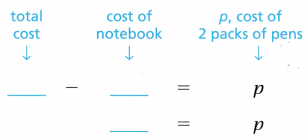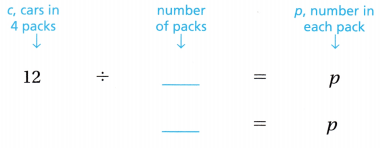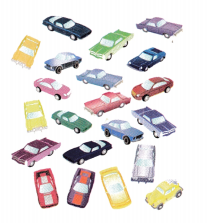# Texas Go Math Grade 3 Lesson 13.5 Answer Key Two-Step Problems

Refer to our Texas Go Math Grade 3 Answer Key Pdf to score good marks in the exams. Test yourself by practicing the problems from Texas Go Math Grade 3 Lesson 13.5 Answer Key Two-Step Problems.

## Texas Go Math Grade 3 Lesson 13.5 Answer Key Two-Step Problems

Essential Question
How con you use the strategy oct it out to solve two-step problems?

Unlock the Problem
Madilyn bought 2 packs of pens and a notebook for $11. The notebook cost$3. Each pack of pens cost the same amount. What is the price of 1 pack of pens?

What do I need to find?
I need to find the price of
1 pack of ___.

What information am I given?
She bought ___ packs of pens and ___ notebook.
The notebook cost ___.

Plan
What is my plan or strategy?
I will use the information to ___ out the problem.

Solve
Describe how to act out the problem.Now I know that 2 packs of pens cost ___.
Next, make an array with the 8 remaining counters to show the cost of the 2 packs of pens.
Write an equation.
$8 ÷ __ = ___ So, the price of 1 pack of pens is ___. Math Talk Mathematical Processes Why do you need to use two operations to solve the problem? Explain. Try Another Problem Chad bought 4 packs of T-shirts. He gave 5 T-shirts to his brother. Now Chad has 19 shirts. How many T-sJiirts were in each pack? Read What do I need to find? What information am I given? Plan What is my plan or strategy? Solve Describe how to act out the problem. • How can you use multiplication and subtraction to check your answer? Math Talk Mathematical processes Explain another strategy you could use to solve this problem. Unlock the Problem Tips ✓ Circle the question. ✓ Underline the important facts. ✓ Choose a strategy you know. Share and Show Question 1. Mac bought 4 packs of toy cars. Then his friend gave him 9 cars. Now Mac has 21 cars. How many cars were in each pack? First, subtract the cars Mac’s friend gave him.Then, divide to find the number of cars in each pack.So, there were ___ cars in each pack. Question 2. What if Mac bought 8 packs of cars and then he gave his friend 3 cars? Mac now has 13 cars. How many cars were in each pack? ___ Answer: Problem Solving Question 3. H.O.T. Evaluate Ryan gave 7 of his model cars to a friend. Then he bought 6 more cars. Now Ryan has 13 cars. How many cars did Ryan start with? ___Answer: Texas Go Math Grade 3 Lesson 13.5 Answer Key Question 4. Multi-Step Chloe bought 5 sets of books. She donated 9 books. Now she has 26 books. How many books were in each set? What strategy did you use? Answer: Daily Assessment Task Fill in the bubble for the correct answer choice. Use a problem-solving model to help you solve. Question 5. There are 32 girls in the Creekview Camping Club. They invite 17 more girls on a camping trip. If they camp at a site with 7 cots in each cabin, how many cabins will they fill? (A) 49 (B) 17 (C) 32 (D) 7 Answer: Question 6. Evaluate Mr. Robb buys 3 packs of socks and a belt for$20. The belt costs $8. If each pack of socks costs the same amount, what is the price of 1 pack of socks? (A)$4
(B) $2 (C)$7
(D) $3 Answer: Question 7. Multi-Step Teanna has 2 boxes of color pencils. One box has 20 color pencils and the other box has 16 color pencils. She gives her brother 3 of the color pencils. She wants to put the color pencils that she has left into 3 equal groups. How many color pencils can Teanna put in each group? (A) 3 (B) 11 (C) 6 (D) 12 Answer: Texas Test Prep Question 8. Mr. Acosta bought 3 packs of markers. Each pack had the same number of markers. He gave 4 markers to his daughter. Now he has 14 markers. How many markers were in each pack? (A) 6 (B) 11 (C) 26 (D) 14 Answer: ### Texas Go Math Grade 3 Lesson 13.5 Homework and Practice Answer Key Problem Solving Question 1. Mr. Tran made a list of some items he sells in his store. He has 5 umbrellas, 2 beach balls, 12 beach chairs, and 9 sunglasses. There are some beach towels stacked equally on two shelves. Mr. Tran determined that there are 46 items. How many beach towels are on each shelf? Describe how to solve the problem. Answer: Question 2. Nickolas spends$17 to buy 4 cartons of yogurt and 3 bottles of juice. If each carton of yogurt costs $2, how much does each bottle of juice cost? Explain. Answer: Question 3. Karina has 55 marbles sorted equally into 5 bags. She gives her friend 10 marbles by pulling them equally from each bag. How many marbles does have now in each of her 5 bags? Write equations to solve. Answer: Question 4. Roberto buys 6 snack packages for his dogs. Each package has 4 snacks. He uses all of the snacks to give 3 snacks to each of his dogs. How many dogs does Roberto have? Answer: Question 5. Melinda has a collection of 54 seashells. She find 27 more shells. If Melinda wants to display her shells equally on 9 shelves, how many shells will she put on each shelf? Write equations to solve. Answer: Lesson Check Fill in the bubble completely to show your answer. Question 6. Multi-Step There are 22 adults and 41 children at a picnic. They sit at picnic tables in equal groups of 9. How many picnic tables are used? (A) 8 (B) 7 (C) 9 (D) 6 Answer: Question 7. Multi-Step Craig buys 4 bags of dog treats for$3 each. He also buys 6 bags of cat treats for $4 each. How much money does Craig spend? (A)$7
(B) $24 (C)$12
(D) $36 Answer: Question 8. Multi-Step Drew has 2 boxes of oatmeal. Each box has 9 packages of oatmeal in it. Drew cooks 2 packages of oatmeal every day. How many days will the oatmeal last? (A) 10 (B) 13 (C) 18 (D) 9 Answer: Question 9. Multi-Step Mrs. Johnson bought her nephews 30 pairs of socks. The socks are sold with three pairs in a package. If she gives two packages to each nephew, how many nephews is she giving socks to? (A) 30 (B) 5 (C) 10 (D) 8 Answer: Question 10. Multi-Step Arnie buys 5 cartons of yogurt and 1 gallon of milk for$21. The milk costs $6. How much does each carton of yogurt cost? (A)$12
(B) $5 (C)$3
(D) \$15